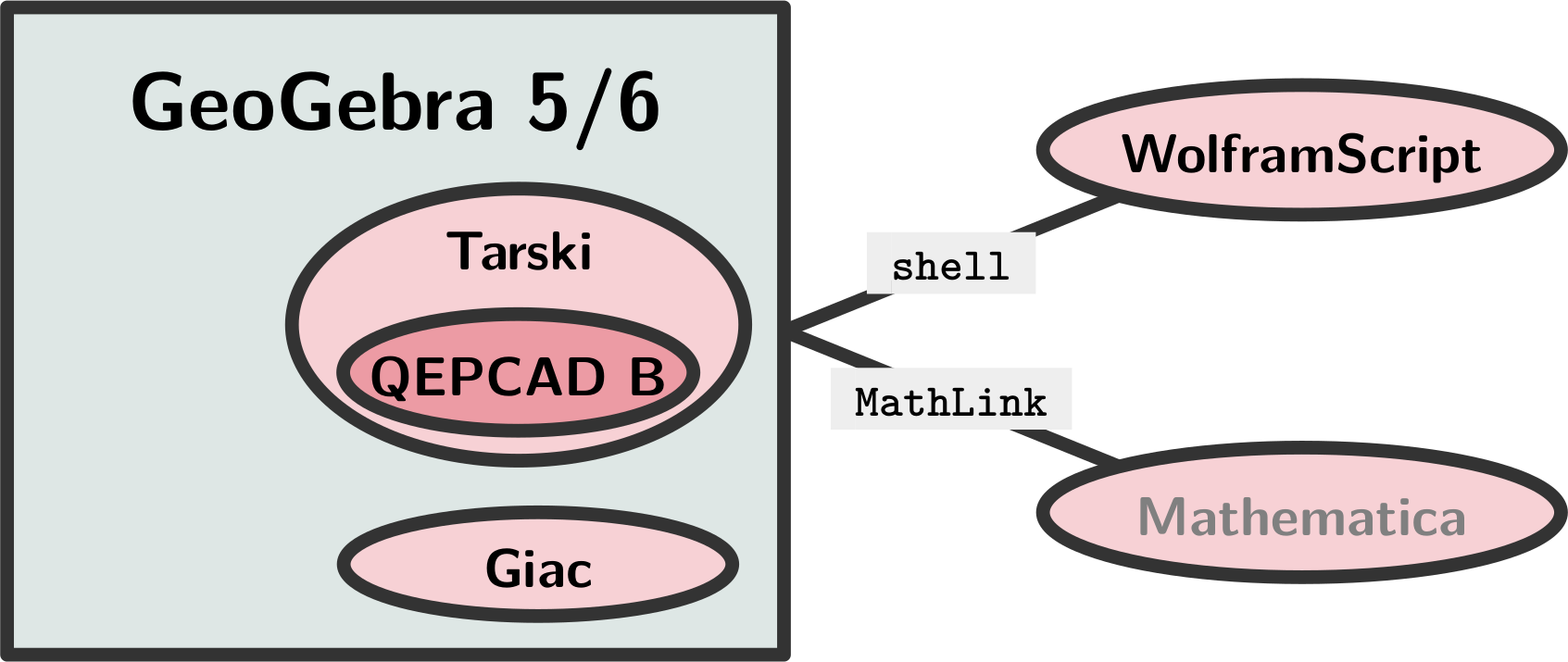# 1 October 2021

## Web version of Tarski

In the previous blog entry three months ago three goals were set. My greatest pleasure is to announce that two of those goals are completed!

In a nutshell: GeoGebra's future versions may require an internal method to symbolically solve a system of inequalities, and eventually also elimination of quantifiers. Meanwhile hard work was dedicated to this project during the summer, however, most of the work was just a kind of dissemination what we plan to do.This figure was created for the ADG 2021 conference, more precisely, for our joint talk with Chris Brown and Róbert Vajda. Now we are happy to announce that this goal has been partially achieved: Tarski is ready to work either as a dynamic library (a DLL) so it can be immediately accessed from a Java application. Also, a web version is ready to go, and this goal was completed with the Emscripten toolchain.

Tarski's syntax may be a bit strange for the first look. It uses a LISP-like input format with nested parentheses. Commands can be given as first words after a parenthesis opens. In our example the command qepcad-api-call is used which means quantifier elimination via an internal API call, namely QEPCAD B. (Luckily, you do not have to know anything about this other tool. It has an even stranger syntax, so better to keep some distance.) After this command a quantified formula is given, here $$\exists x:ax^2+bx+c=0$$.

If things work as expected, after clicking in the pink area and pressing ENTER will give you some output that can be interpreted as $4 a c - b^2 \le 0 \wedge (b \neq 0 \vee c = 0 \vee 4 a c - b^2 < 0).$ So what is the meaning of all of this?

We ask the question: For which $$x$$ values does the quadratic equation $$ax^2+bx+c=0$$ have a solution? Answer: If $$a\neq0$$, there is a solution if and only if the discriminant satisfies $$b^2-4ac\ge0$$. Otherwise, the equation is not quadratic, and in that case there are two subcases: If $$b\ne0$$, a solution always exists; otherwise $$c$$ must also be 0. It is quite clear that Tarski gave an equivalent answer here.

We note that Tarski actually eliminated the existential quantifier. For this step some sophisticated algorithms were required in the background. Here we do not go into the detail: the magic words are cylindrical algebraic decomposition and real quantifier elimination.

Due to our best knowledge there is no other web based system that can compute such questions to date!

### Some technical background

We extended Tarski to be able to compile with Emscripten. To achieve this the source code had to be edited at some places where Emscripten did not support certain C++ techniques. We also compiled openssl for Emscripten and copied in its system folder. After then, the command line TOOLCHAIN=emmake ./build.sh successfully compiles the WebAssembly version of Tarski, and three files are provided: an .html wrapper, a .js interface and the .wasm code.

Here you can download the most recent binaries for various platforms (including WebAssembly, of course).

### More examples

Maybe you want to try out some examples that are known to work in the web version:
• (qepcad-api-call [ ex x [ x>0 ] ] )
• (Is there a positive number?)
• (qepcad-api-call [ ex x [ x^2<0 ] ] )
• (Is there a number such that its square is negative?)
• (t-neg [all m[ex x[x^2>m]]])
• (What is the negation of the statement that the function $$x^2$$ has no upper bound?)Zoltán Kovács Linzer Zentrum für Mathematik Didaktik Johannes Kepler Universität Altenberger Strasse 54 A-4040 Linz